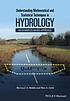# Classify

## Summary

Title:
Understanding mathematical and statistical techniques in hydrology : an examples-based approach
Author:
Rodda, Harvey; Little, Max A.
Formats:

Editions:
16
Total Holdings:
297
OCLC Work Id:
2549410452
http://classify.oclc.org/classify2/ClassifyDemo?owi=2549410452
Most Frequent 551.480151 267 Web Dewey
Edition: 23 551.480151 258 Web Dewey
Most Frequent GB656.2.M33 285 ClassWeb## Editions

Displaying 1 to 16 of 16
Title and AuthorLanguageFormatHoldings TagClass NumberUnderstanding mathematical and statistical techniques in hydrology : an examples-based approachby Rodda, Harveyeng 193082551.480151

(DDC 23)

050GB656.2.M33Understanding mathematical and statistical techniques in hydrology : an examples-based approachby Rodda, Harveyeng 41082551.480151

(DDC 23)

050GB656.2.M33Understanding Mathematical and Statistical Techniques in Hydrology : An Examples-based Approachby Rodda, Harveyeng 19050GB656.2.M33
082510Understanding mathematical and statistical techniques in hydrology : an examples-based approachby Rodda, Harveyeng 9082551.480151
050GB656.2.M33Understanding mathematical and statistical techniques in hydrology : an examples-based approachby Rodda, Harveyeng 8050GB656.2.M33
082551.480151

(DDC 23)Understanding mathematical and statistical techniques in hydrology : an examples-based approachby Rodda, Harveyeng 6082551.480151

(DDC 23)

050GB656.2.M33Understanding mathematical and statistical techniques in hydrology : an examples-based approachby Rodda, Harveyeng 5082551.480151

(DDC 23)

050GB656.2.M33Understanding mathematical and statistical techniques in hydrology : an examples-based approachby Rodda, Harveyeng 5Understanding mathematical and statistical techniques in hydrology : an examples-based approachby Rodda, Harveyeng 3082551.480151

(DDC 23)

050GB656.2.M33Understanding Mathematical and Statistical Techniques in Hydrology : An Examples-based Approachby Rodda, Harveyeng 2082550Understanding mathematical and statistical techniques in hydrology : an examples-based approachby Rodda, Harveyeng 1082551.480151

(DDC 23)UNDERSTANDING MATHEMATICAL AND STATISTICAL TECHNIQUES IN HYDROLOGY : an examples-based ... approachby Rodda, Harveyeng 1Understanding mathematical and statistical techniques in hydrology : an exemples-based approachby Rodda, Harveyeng 1Understanding Mathematical and Statistical Techniques in Hydrology : An Examples-Based Approachund 1Understanding mathematical and statistical techniques in hydrology : an examples-based approachby Rodda, Harveyeng 1Understanding mathematical and statistical techniques in hydrology : an examples-based approachby Rodda, Harveyeng 1082551.480151

(DDC 23)

050GB656.2.M33
Displaying 1 to 16 of 16
.